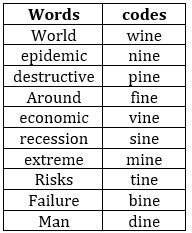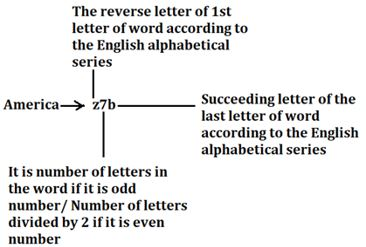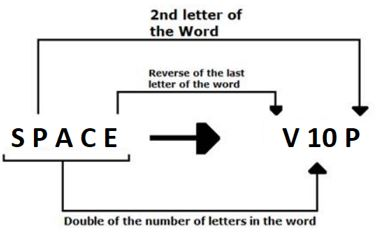Latest Banking jobs   »

# Reasoning Ability Quiz For SIDBI GRADE A 2023- 10th January

Direction (1-5): Study the following information carefully and answer the questions given below:
In a certain code language:
‘Destructive epidemic around world’ is coded as ‘pine fine nine wine’
‘World extreme economic recession’ is coded as ‘mine vine sine wine’
‘Epidemic risks economic failure’ is coded as ‘nine tine vine bine’
‘Man risks destructive recession’ is coded as ‘pine dine tine sine’

Q1. What is the code of “recession” as per the given code language?
(a) mine
(b) sine
(c) dine
(d) wine
(e) None of these

Q2. What may be the possible code of “destructive cyclone” as per the given code language?
(a) mine fine
(b) sine pine
(c) pine line
(d) wine sine
(e) mine pine

Q3. What is the code of “economic risks” as per the given code language?
(a) vine tine
(b) sine vine
(c) dine tine
(d) wine dine
(e) None of these

Q4. Which of the following word is coded as “nine” as per the given code language?
(a) world
(b) recession
(c) extreme
(d) epidemic
(e) None of these

Q5. What is the code of “extreme” as per the given code language?
(a) sine
(b) mine
(c) dine
(d) wine
(e) None of these

Direction (6-10): Study the following information carefully and answer the questions given below:
In a certain code language:
‘China America conducts meeting’ is coded as ‘x5b z7b x4t n7h’
‘Online session for students’ is coded as ‘l3f h7o u3s h4t’
‘Masks sale growing fast’ is coded as ‘n5t h2f t7h u2u’
‘School and college closed’ is coded as ‘h3m z3e x7f x3e’

Q6. What is the code of ‘escape’ as per the given code language?
(a) v6f
(b) v3f
(c) f6v
(d) f3v
(e) None of these

Q7. What is the code of ‘migrant worker’ as per the given code language?
(a) n7u d3s
(b) m7u d6s
(c) n8u w3r
(d) m8t w6s
(e) None of these

Q8. Which of the following word is coded as ‘t5t’ as per the given code language?
(a) Gathering
(b) Greetings
(c) Terrible
(d) Transport
(e) Guidelines

Q9. What is the code of ‘immunity’ as per the given code language?
(a) r8z
(b) r4y
(c) i4z
(d) i8y
(e) None of these

Q10. Which of the following pair of words is coded as ‘self isolation’ as per the given code language?
(a) h2g i9o
(b) h4g i9o
(c) h2g r9o
(d) s4g r9n
(e) s2g r9n

Direction (11-15): Study the following information carefully and answer the following question.
The following words are coded in this manner:
“Hit Two Golf Ball” is coded as “O8A U8O G6I L6W”
“You Wax Inner Space” is coded as “F6O I10N C6A V10P”
“Part Centre Control Fixed” is coded as “G8A V12E O14O W10I”

Q11. What is the code for ‘Reverse’?
(a) S10E
(b) V14R
(c) R14S
(d) V14E
(e) None of these

Q12. What is the code for ‘Immediately’?
(a) Y22I
(b) L24M
(c) B24M
(d) B22M
(e) None of these

Q13. ‘V18A’ is a code from which word?
(a) Wanted
(b) Sartre
(c) Backspace
(d) Reynolds
(e) None of these

Q14. What is the code for ‘Every Fact’?
(a) B12U G6A
(b) B10V G8A
(c) H12D J8B
(d) F8T U10V
(e) None of these

Q15. ‘G14L V12X’ is the code for which word?
(a) Elantra Bank
(b) Electric Break
(c) Electra Example
(d) Element Expose
(e) None of these

Solutions

Solutions (1-5):
Sol.S1. Ans. (b)
S2. Ans. (c)
S3. Ans. (a)
S4. Ans. (d)
S5. Ans. (b)

Direction (6-10):
Sol.S6. Ans. (b)
S7. Ans. (a)
S8. Ans. (e)
S9. Ans. (e)
S10. Ans. (c)

Solutions (11-15):
Sol. This logic will be followed in all words:S11. Ans. (d)
S12. Ans. (d)
S13. Ans. (c)
S14. Ans. (b)
S15. Ans. (d)## FAQs

### When will the SIDBI Grade A Exam 2023 be held?

The SIDBI Grade A Exam 2023 will be held on 28th January 2023.

#### Congratulations!